# 9.1 Tracking 2D Orientation

This section explains how the orientation of a rigid body is estimated using an inertial measurement unit (IMU). The main application is determining the viewpoint orientation,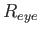from Section 3.4, while the user is wearing a VR headset. Another application is estimating the orientation of a hand-held controller. For example, suppose we would like to make a laser pointer that works in the virtual world, based on a direction indicated by the user. The location of a bright red dot in the scene would be determined by the estimated orientation of a controller. More generally, the orientation of any human body part or moving object in the physical world can be determined if it has an attached IMU.

To estimate orientation, we first consider the 2D case by closely following the merry-go-round model of Section 8.1.2. The technical issues are easy to visualize in this case, and extend to the more important case of 3D rotations. Thus, imagine that we mount a gyroscope on a spinning merry-go-round. Its job is to measure the angular velocity as the merry-go-round spins. It will be convenient throughout this chapter to distinguish a true parameter value from an estimate. To accomplish this, a hat'' will be placed over estimates. Thus, let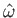correspond to the estimated or measured angular velocity, which may not be the same as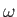, the true value.

How areandrelated? If the gyroscope were functioning perfectly, thenwould equal; however, in the real world this cannot be achieved. The main contributor to the discrepancy betweenandis calibration error. The quality of calibration is the largest differentiator between an expensive IMU (thousands of dollars) and cheap one (a dollar).

We now define a simple model of calibration error. The following sensor mapping indicates how the sensor output is related to true angular velocity: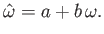(9.1)

Above,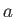and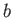are called the offset and scale, respectively. They are unknown constants that interfere with the measurement. Ifwere perfectly measured, then we would have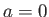and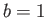.

Consider the effect of calibration error. Comparing the measured and true angular velocities yields: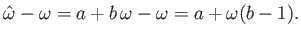(9.2)

Now imagine using the sensor to estimate the orientation of the merry-go-round. We would like to understand the difference between the true orientation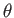and an estimate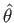computed using the sensor output. Let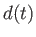denote a function of time called the drift error: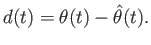(9.3)

Note thatmight be negative, which could be forced into being positive by applying the absolute value to obtain. This will be avoided to simplify the discussion.

Suppose it is initially given that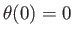, and to keep it simple, the angular velocityis constant. By integrating (9.2) over time, drift error is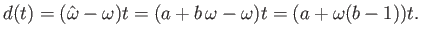(9.4)

Of course, the drift error grows (positively or negatively) asdeviates from 0 or asdeviates from one; however, note that the second component is proportional to. Ignoring, this means that the drift error is proportional to the speed of the merry-go-round. In terms of tracking a VR headset using a gyroscope, this means that tracking error increases at a faster rate as the head rotates more quickly .

At this point, four general problems must be solved to make an effective tracking system, even for this simple case:

1. Calibration: If a better sensor is available, then the two can be closely paired so that the outputs of the worse sensor are transformed to behave as closely to the better sensor as possible.
2. Integration: The sensor provides measurements at discrete points in time, resulting in a sampling rate. The orientation is estimated by aggregating or integrating the measurements.
3. Registration: The initial orientation must somehow be determined, either by an additional sensor, or a clever default assumption or start-up procedure.
4. Drift error: As the error grows over time, other sensors are needed to directly estimate it and compensate for it.
All of these issues remain throughout this chapter for the more complicated settings. The process of combining information from multiple sensor readings is often called sensor fusion or filtering.

We discuss each of these for the 2D case, before extending the ideas to the 3D case in Section 9.2.

Subsections
Steven M LaValle 2019-03-14